# Inter maths solutions for IIA Partial Fractions

Intermediate second year mathematics text book chapter  exercises 7(a, 7(b), 7(c) and 7(d) solutions are given.

These solutions are very easy to understand. Study the text book lesson Partial Fractions very well.

Observe the example problems and solutions given in the textbook.

Observe the solutions and try them in your own method.

You can also see
Inter Maths 1A textbook solutions

Inter Maths 1B textbook solutions

Inter Maths IIA textbook solutions

Inter Maths IIB textbook solutions

M

# Exercise 7(a) 7(b) 7(c) 7(d) Intermediate 2nd year Mathematics solutions

## PARTIAL FRACTIONS solutions

Chapter – 7

Partial Fractions – EXERCISE 7(a)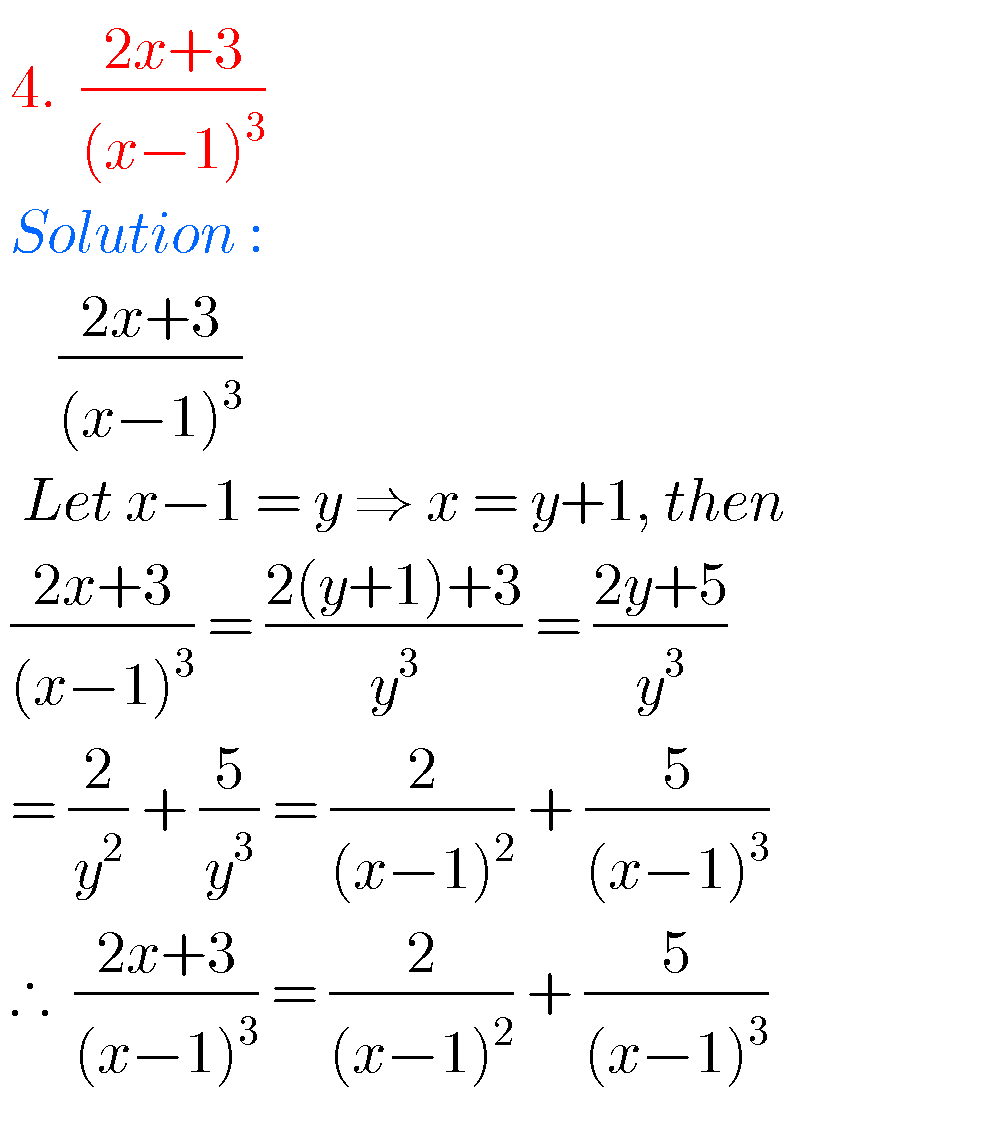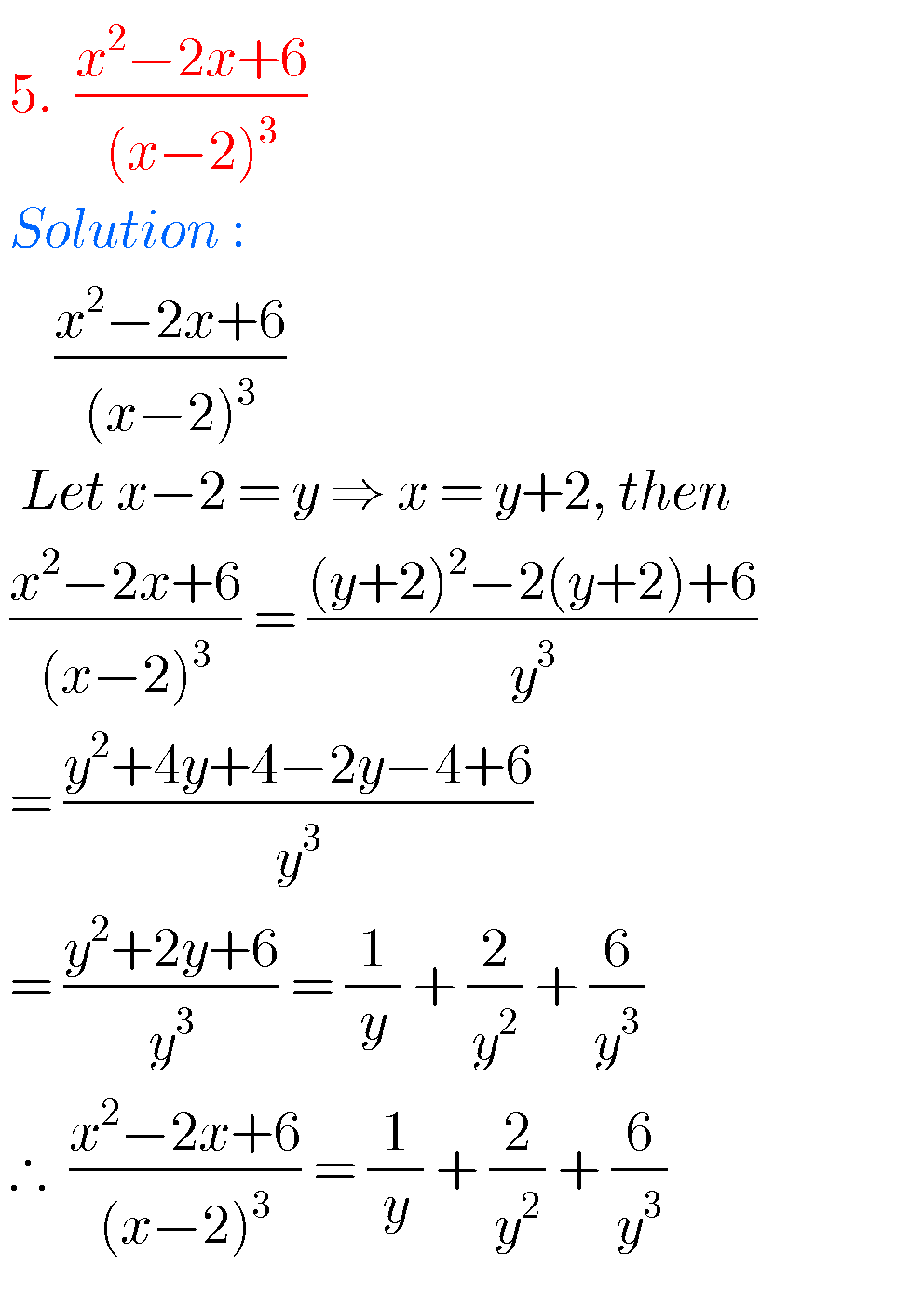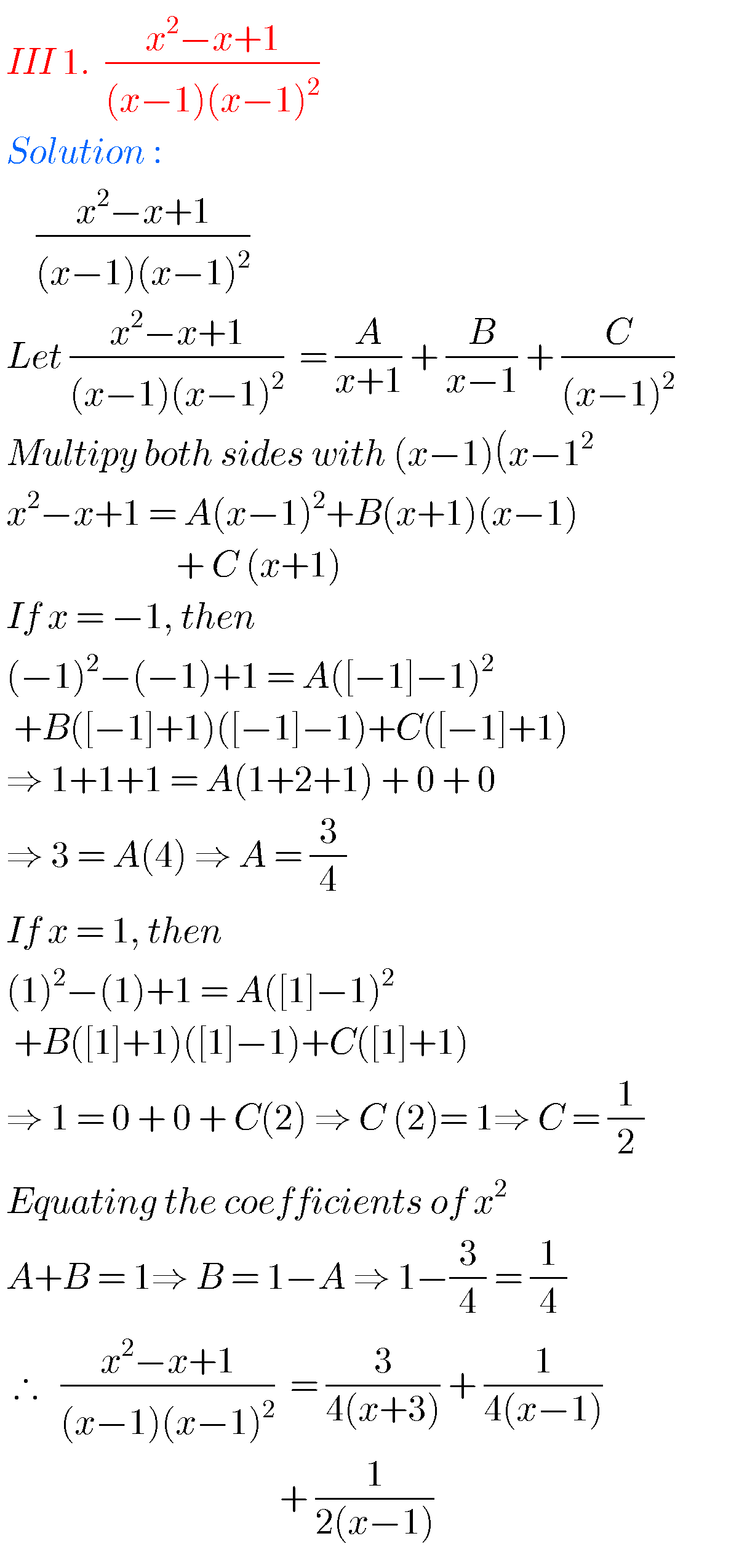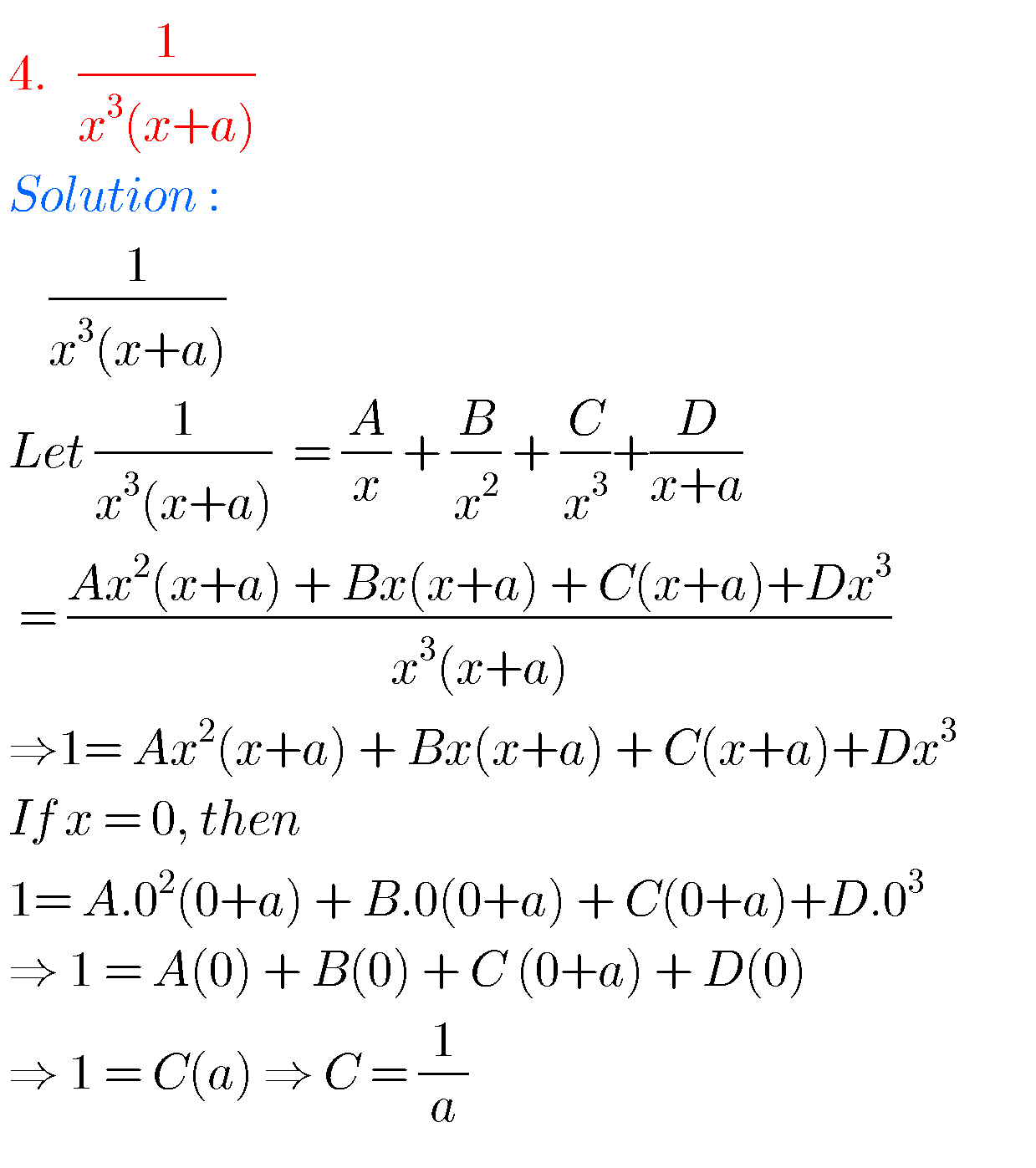## Partial Fractions solutions Inter

Partial Fractions – EXERCISE  7(b)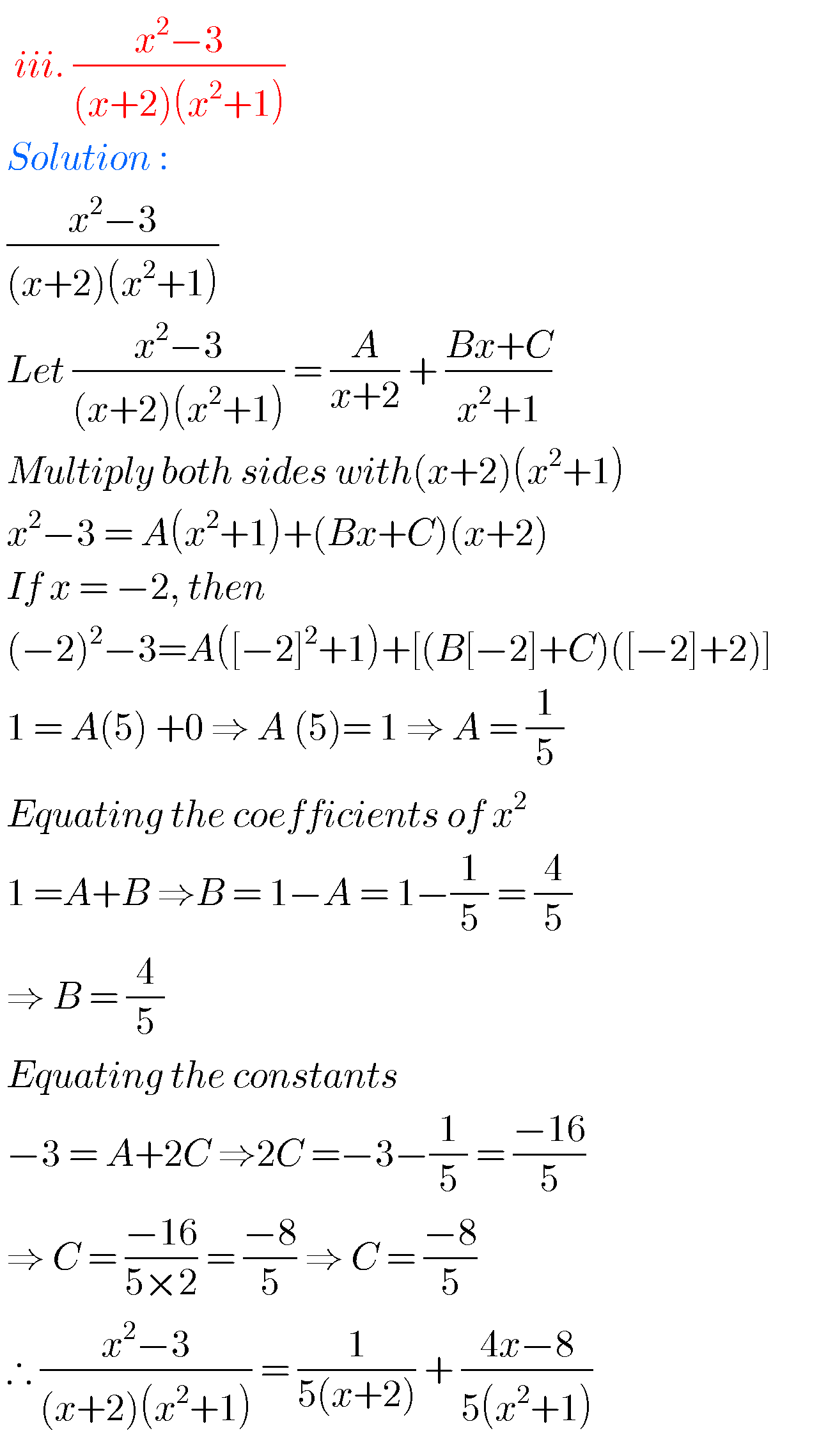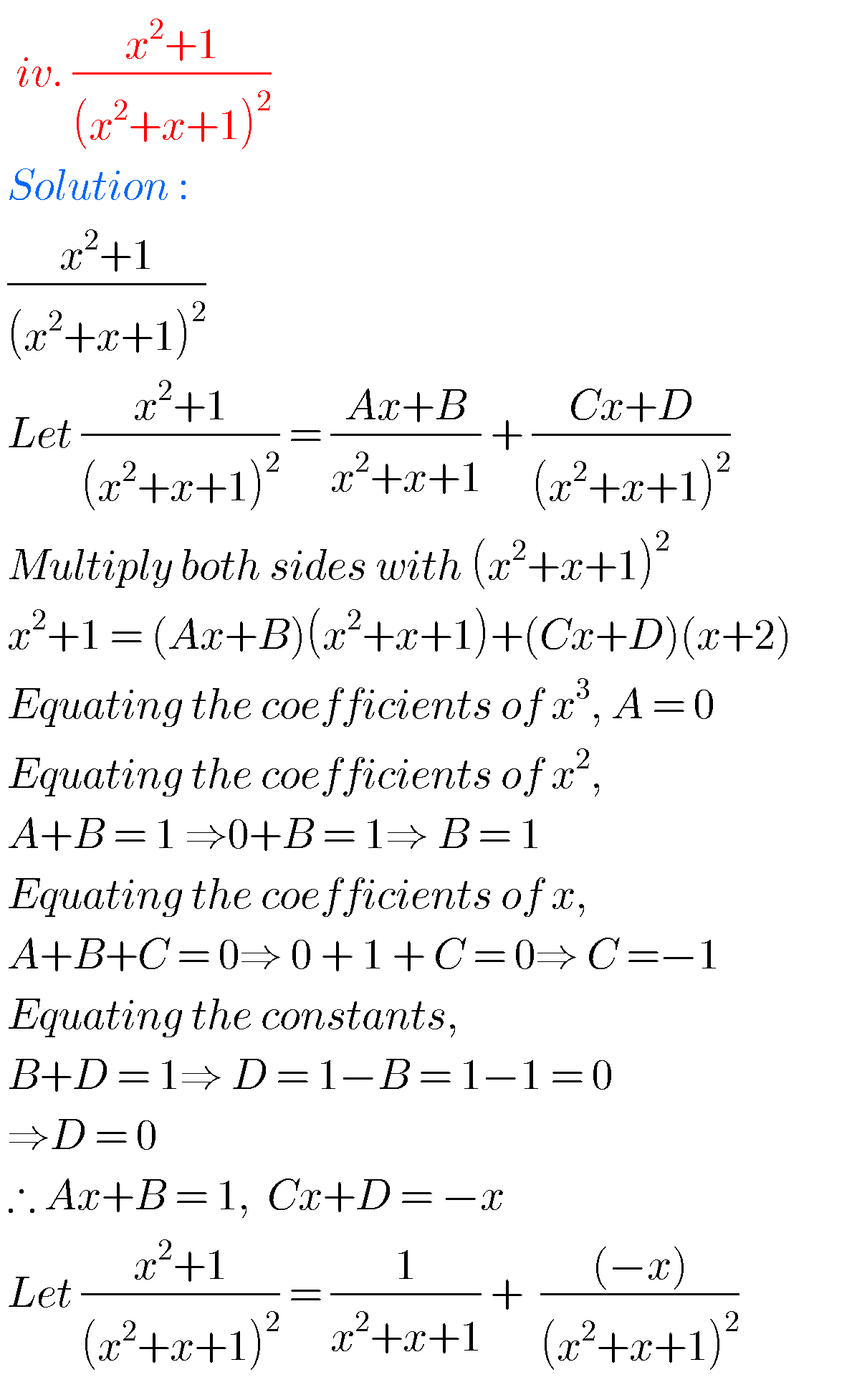### Text book exercises solutions for partial fractions inter

Partial Fractions – EXERCISE 7(c)### Maths Inter 2a solutions for chapter 7

Partial Fractions – EXERCISE 7(d)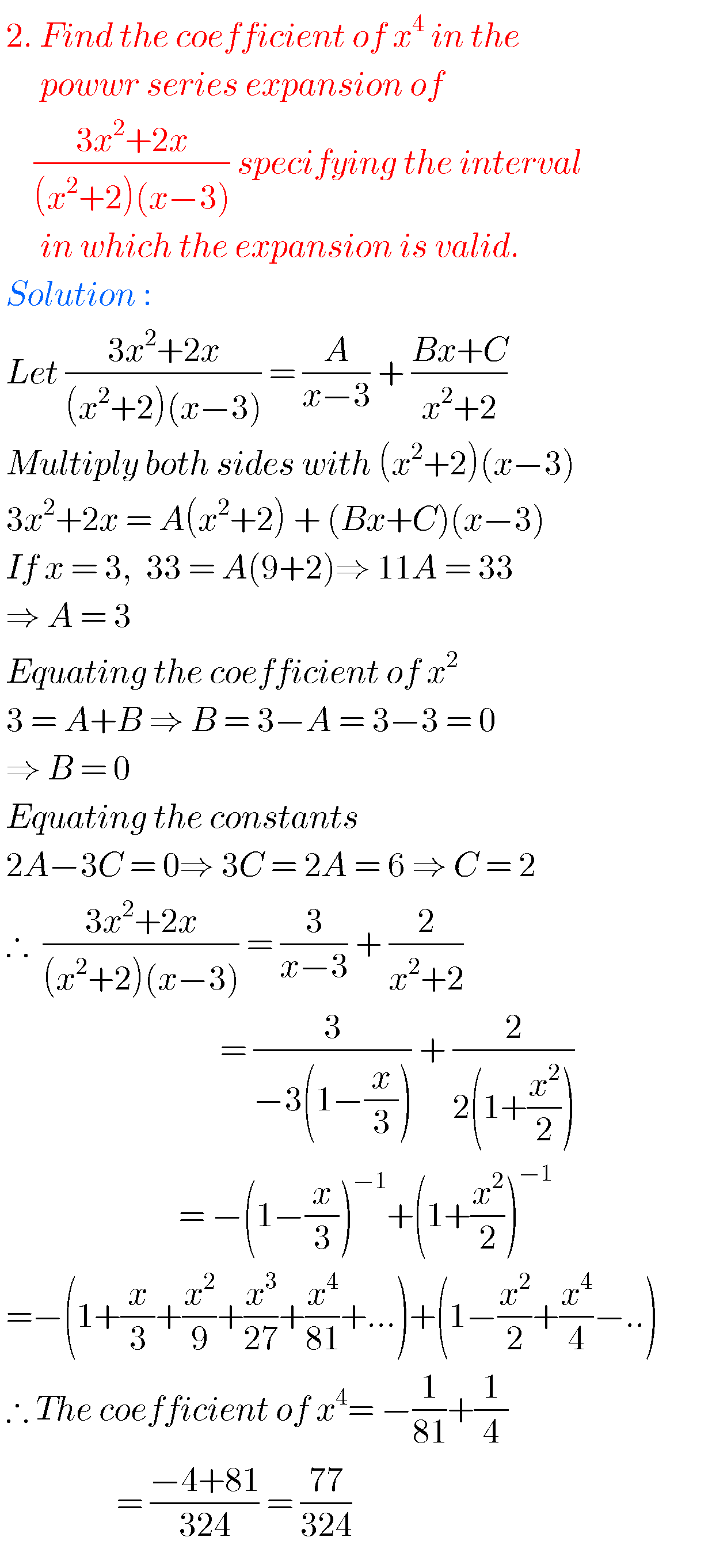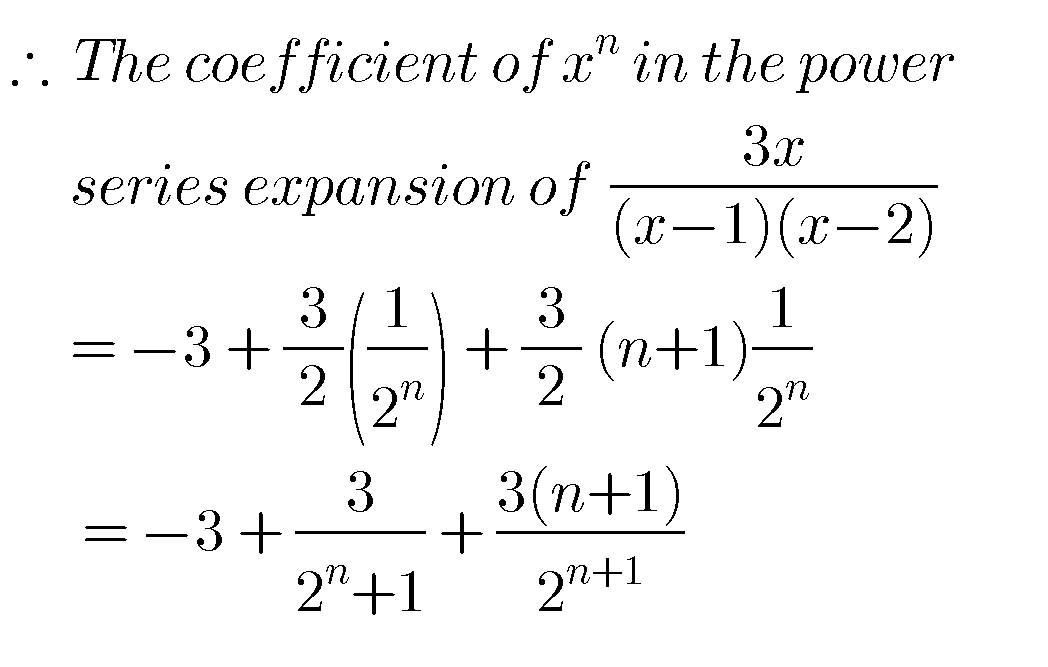Note : Observe the solutions and try them in your own methods.

class 10 maths polynomials solutions

Ncert maths class 8 exponents and powers

Ncert maths class 7 fractions and decimals

Ncert maths class 6 playing with numbers

### 2 thoughts on “Inter maths solutions for IIA Partial Fractions,exercise 7(a) 7(b) 7(c) 7(d) Intermediate Mathematics 2nd year solutions”

1.Thanks you sir

2.Best application I am searching it for soo many days it is the best I have seen ,keep it up guys I am soo happy for this